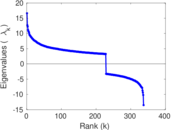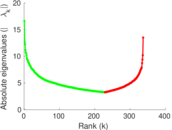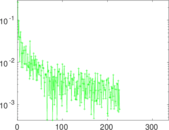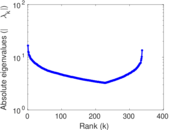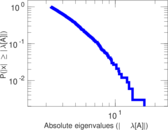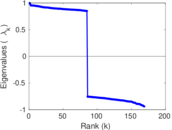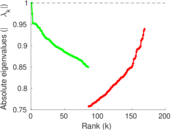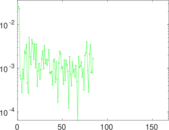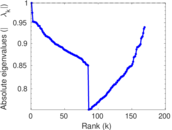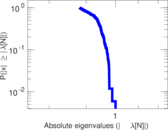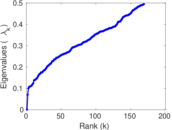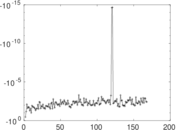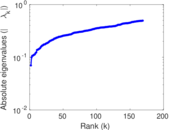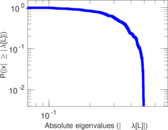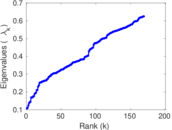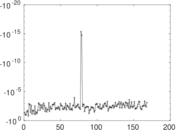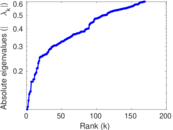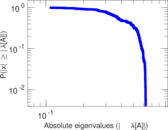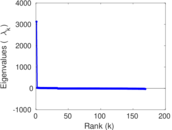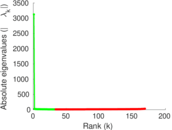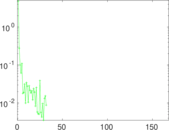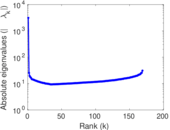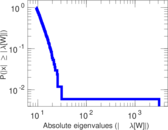# Human proteins (Vidal)

This network represens an initial version of a proteome-scale map of Human binary protein–protein interactions

 Code `MV` Internal name `maayan-vidal` Name Human proteins (Vidal) Data source http://research.mssm.edu/maayan/datasets/qualitative_networks.shtml AvailabilityDataset is available for download Consistency checkDataset passed all tests Category Metabolic network Node meaning Protein Edge meaning Interaction Network format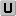Unipartite, undirected Edge typeUnweighted, no multiple edges Loops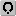Contains loops

## Statistics

 Size n = 3,133 Volume m = 6,726 Loop count l = 577 Wedge count s = 88,809 Claw count z = 1,399,841 Cross count x = 27,308,384 Triangle count t = 1,047 Square count q = 10,216 4-Tour count T4 = 449,262 Maximum degree dmax = 129 Average degree d = 4.293 65 Fill p = 0.001 370 02 Size of LCC N = 2,783 Diameter δ = 13 50-Percentile effective diameter δ0.5 = 4.264 35 90-Percentile effective diameter δ0.9 = 5.872 22 Median distance δM = 5 Mean distance δm = 4.803 13 Gini coefficient G = 0.540 534 Balanced inequality ratio P = 0.294 826 Relative edge distribution entropy Her = 0.926 225 Power law exponent γ = 2.114 32 Tail power law exponent γt = 3.031 00 Tail power law exponent with p γ3 = 3.031 00 p-value p = 0.882 000 Degree assortativity ρ = −0.125 658 Degree assortativity p-value pρ = 1.817 28 × 10−44 Clustering coefficient c = 0.035 368 0 Spectral norm α = 16.614 4 Algebraic connectivity a = 0.069 808 5 Spectral separation |λ1[A] / λ2[A]| = 1.229 05 Non-bipartivity bA = 0.186 364 Normalized non-bipartivity bN = 0.060 887 7 Algebraic non-bipartivity χ = 0.107 163 Spectral bipartite frustration bK = 0.005 790 50 Controllability C = 820 Relative controllability Cr = 0.261 730

## Plots

### Fruchterman–Reingold graph drawing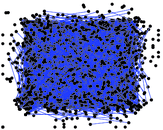### Degree distribution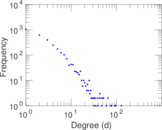### Cumulative degree distribution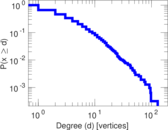### Lorenz curve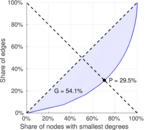### Spectral distribution of the adjacency matrix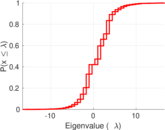### Spectral distribution of the normalized adjacency matrix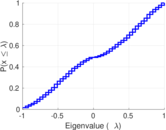### Spectral distribution of the Laplacian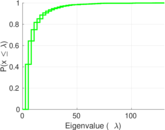### Spectral graph drawing based on the adjacency matrix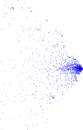### Spectral graph drawing based on the Laplacian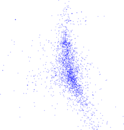### Spectral graph drawing based on the normalized adjacency matrix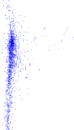### Degree assortativity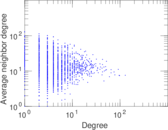### Zipf plot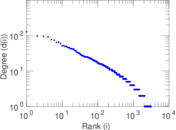### Hop distribution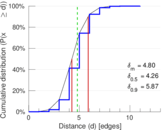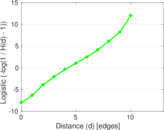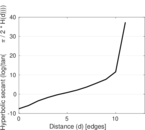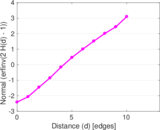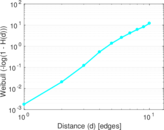### Double Laplacian graph drawing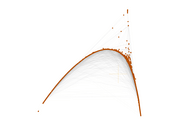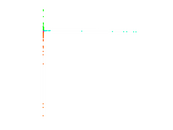### Delaunay graph drawing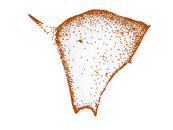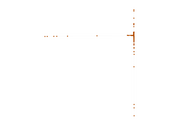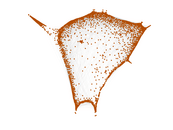### Clustering coefficient distribution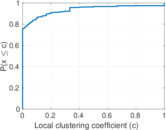### Average neighbor degree distribution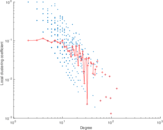### SynGraphy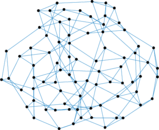### Matrix decompositions plots